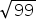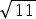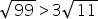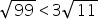Mathematics
Easy

Question

# Compareto 3.

## EquivalentNot equivalentHint:

## The correct answer is: Equivalent

### Here, we have to compare √99 and 3√11.We have, √99 = √(3 × 3 × 11) . = 3√11 .Clearly, we can see √99 is equal to 3√11 . Therefore, these are equivalent fractions.Hence, the correct option is (A).

The values of equivalent expressions are the same.

### Related Questions to study#### With Turito Foundation.#### Get an Expert Advice From Turito.